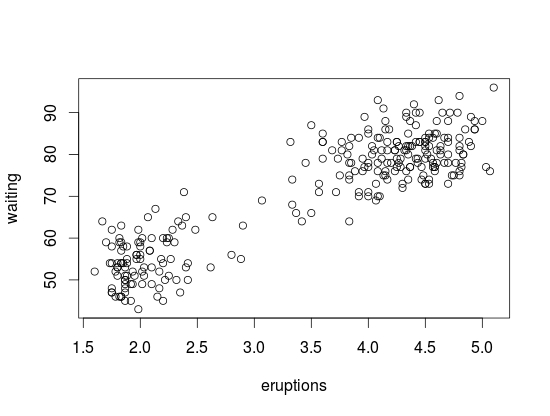# Intro to Making Plots in R

One of the strongest points of R in the opinion of many are the various features for creating graphs and other visualizations of data. In this post, we begin to look at using the various visualization features of R. Specifically, we are going to do the following

• Using data in R to display graph
• Add text to a graph
• Manipulate the appearance of data in a graph

Using Plots

The ‘plot’ function is one of the basic options for graphing data. We are goingto go through an example using the ‘islands’ data that comes with the R software. The ‘islands’ software includes lots of data, in particular, it contains data on the lass mass of different islands. We want to plot the land mass of the seven largest islands. Below is the code for doing this.

`islandgraph<-head(sort(islands, decreasing=TRUE), 7)`
`plot(islandgraph, main = "Land Area", ylab = "Square Miles")`
`text(islandgraph, labels=names(islandgraph), adj=c(0.5,1))`

Here is what we did

1. We made the variable ‘islandgraph’
2. In the variable ‘islandgraph’ We used the ‘head’ and the ‘sort’ function. The sort function told R to sort the ‘island’ data by decreasing value ( this is why we have the decreasing argument equaling TRUE). After sorting the data, the ‘head’ function tells R to only take the first 7 values of ‘island’ (see the 7 in the code) after they are sorted by decreasing order.
3. Next, we use the plot function to plot are information in the ‘islandgraph’ variable. We also give the graph a title using the ‘main’ argument followed by the title. Following the title, we label the y-axis using the ‘ylab’ argument and putting in quotes “Square Miles”.
4. The last step is to add text to the information inside the graph for clarity. Using the ‘text’ function, we tell R to add text to the ‘islandgraph’ variable using the names from the ‘islandgraph’ data which uses the code ‘label=names(islandgraph)’. Remember the ‘islandgraph’ data is the first 7 islands from the ‘islands’ dataset.
5. After telling R to use the names from the islandgraph dataset we then tell it to place the label a little of center for readability reasons with the code ‘adj = c(0.5,1).

Below is what the graph should look like.Changing Point Color and Shape in a Graph

For visual purposes, it may be beneficial to manipulate the color and appearance of several data points in a graph. To do this, we are going to use the ‘faithful’ dataset in R. The ‘faithful’ dataset indicates the length of eruption time and how long people had to wait for the eruption. The first thing we want to do is plot the data using the “plot” function.As you see the data, there are two clear clusters. One contains data from 1.5-3 and the second cluster contains data from 3.5-5. To help people to see this distinction we are going to change the color and shape of the data points in the 1.5-3 range. Below is the code for this.

`eruption_time<-with(faithful, faithful[eruptions < 3, ])`
`plot(faithful)`
`points(eruption_time, col = "blue", pch = 24)`

Here is what we did

1. We created a variable named ‘eruption_time’
2. In this variable, we use the ‘with’ function. This allows us to access columns in the dataframe without having to use the \$ sign constantly. We are telling R to look at the ‘faithful’ dataframe and only take the information from faithful that has eruptions that are less than 3. All of this is indicated in the first line of code above.
3. Next, we plot ‘faithful’ again
4. Last, we add the points from are ‘eruption_time’ variable and we tell R to color these points blue and to use a different point shape by using the ‘pch = 24’ argument
5. The results are belowConclusion

In this post, we learned the following

• How to make a graph
• How to add a title and label the y-axis
• How to change the color and shape of the data points in a graph